# Area and volume

## Summary and examples

The picture below shows the graph of the function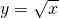on the interval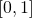(the figure is taken from Wikipedia).Suppose we want to calculate the area enclosed by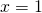,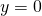and. Because we (still) do not know how to calculate an area with a curved boundary (in this case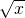) we could opt for the following approach. We approximate the area by adding the areas of the yellow rectangles: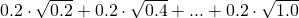In this way we approximate the area 'from above', because the sum of the areas of the yellow rectangles is larger than the area we want to calculate. We could also take the sum of the green rectangles and then we would approximate the area 'from below'.

However, in both cases the approximation is too rough and thus we could choose a smaller width, e.g.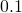. Also this approximation is not good enough and therefore we choose a width equal to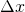with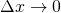. That is to say, we take smaller and smaller values for. Note that taking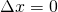yields an area equal to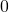and that is certainly not correct. The area can be calculated as follows: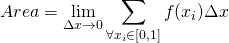In this example we have: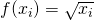For this complicated formula we write for brevity: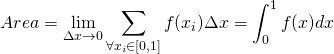This shorthand notation is called a definite integral between the limits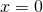and.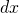is the 'infinitely small' version of the very small version of.
In Integration of standard functions we explain how to calculate this definite integral.

In this section we only consider the calculation of areas and conclude from the above that integration can help us. Later we will see that we can use integrals for volumes as well, especially solids of revolution.
Before we proceed with the details of the calculation of areas by integrating, we just finish the integral above.

In Integration of standard functions we see that the primitive function of: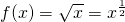equals: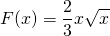and thus: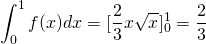We have to make a few remarks. In the above case, the graph of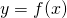and thus the enclosed area lies above the-axis. When the graph of a function graph and thus the enclosed area lie under the-axis the integral would have given a negative result. Foris positive, but the successive values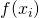would all be negative, and thus the integral.

##### Example 1

Calculate the area enclosed byand the-axis, betweenand.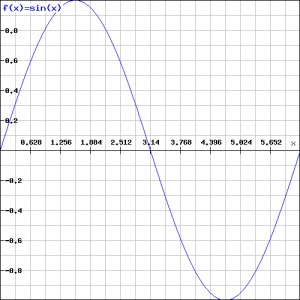We could have calculated this as follows, but the result might surprise us: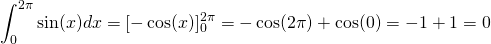Of course, this strange result is not correct. It can be explained by the fact that the 'positive' area above the-axis is compensated by the equal 'negative' area below the-axis.
The correct result  could be obtained as follows: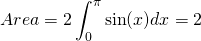##### Example 2

Revolving the graph ofaround the-axis we get a paraboloid (figure from www.math4all.nl). In the figure below we see the surface of revolution, in this case for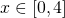.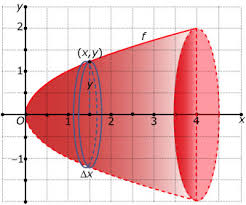How could we calculate the volume of this solid of revolution? We proceed as follows.

Suppose that we draw a circle at a given pointwith radius equal to. Based on this we construct a cylinder of heightand then the volume of this cylinder equals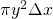. The total volume can be approximated by adding the volumes of all of these small cylinders and next by takingsmaller and smaller::In this case we have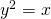and thus we get: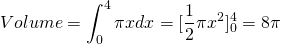When we would have revolved the graph around the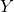-axis, for example betweenand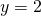, the calculation can be carried out similarly (note that).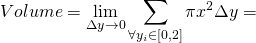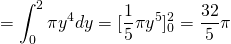##### Example 3

Calculate the volume of the cone with radius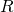and height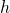. We know from books that the volume is:The cone is constructed by revolving a straight line around the-axis. We should take the following line: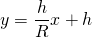and note that this line goes trough the points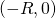and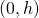. Revolving this line around the-axis yields the cone: a circle with radiusand height.

In order to calculate the volume we use the formula: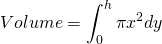Forwe have: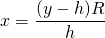and thus: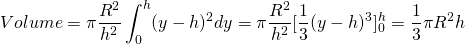0